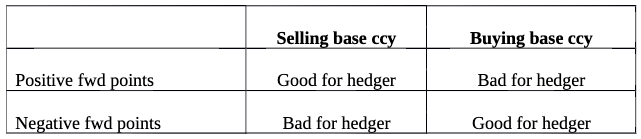Search
•Paul Stafford

# Forward rates, interest rates, and managing risk

Updated: Nov 30, 2020

Forward exchange rates for currencies are a function of the respective interest rates of the base (left side) currency and term (right side) currency. The basic equation for calculating forward rates with the domestic currency as the base currency is:

Forward rate = Spot rate * (1+IR foreign)/(1+IR domestic)

It is not simply the difference!

Forward points may be positive or negative. Whether that is advantageous for a hedger depends on which currency they're selling and which they're buying.For example, USDZAR one year forward points = 8830. Current USDZAR spot = 16.14, giving a forward rate of 16.14 + 8830/10000 = 17.02. If the hedger is selling ZAR forward, he incurs a penalty of 5.5%

Conversely, GBPJPY one year fwd points = -148, GBPJPY spot = 135, and the forward rate is 133.5; less than the spot. If the hedger is selling JPY forward, he benefits by a little over 1%.

For hedgers selling the foreign currency (a common scenario), a reduction in the foreign rate is helpful, reducing the ratio. For hedgers buying foreign currency, a reduction in the domestic rate is helpful.

On March 3rd, the FED reduced the federal funds rate by .5% to about 1%; and the BoE cut by the same amount, from 0.75% to 0.25%. Emerging market central banks are doing the same. The Central Bank of Brazil cut its rate from 5% to 4.5% in December and another 25 bps in Feb to 4.25% (a record low). For perspective, in 2016 the rate was 14.25%. While these rates are not the exact ones used in the equation above, they are closely related.

As central banks race to decrease their rates, converging on zero, the ratios approach 1. This helps all hedgers, as a forward rate close to spot exacts no penalty in either direction. With the markets showing elevated volatility, there is little excuse not to manage risk.